Anzeige

# Understanding excel’s error values

Trainer & Mentor at Ameetz Academy - India um Ameetz Academy - India (www.ameetz.com)
18. Feb 2012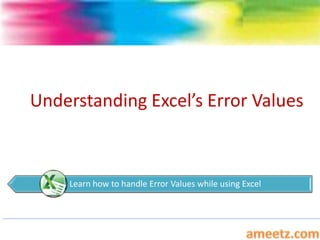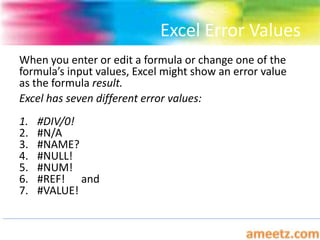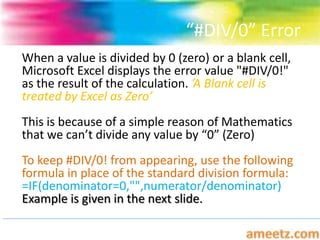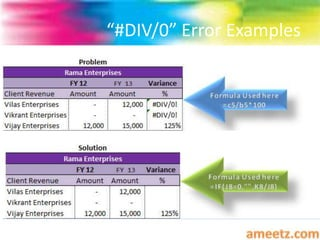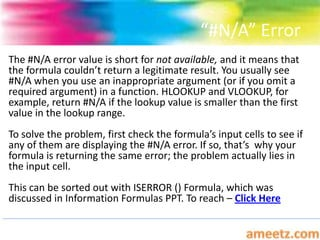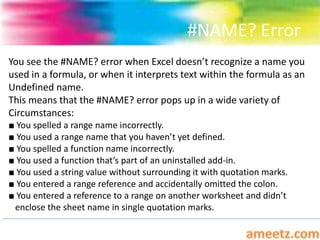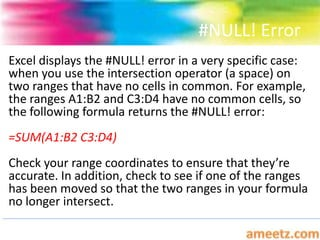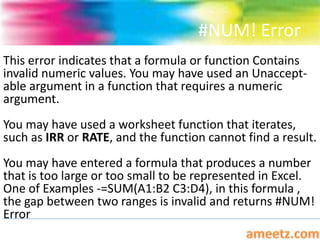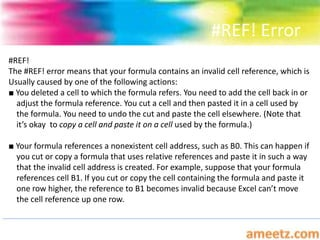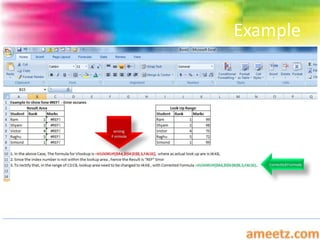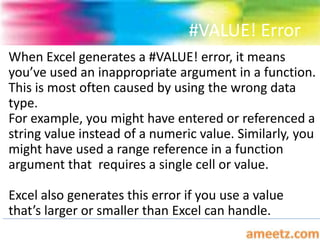1 von 12
Anzeige

### Understanding excel’s error values

1. Understanding Excel’s Error Values Learn how to handle Error Values while using Excel
2. Excel Error Values When you enter or edit a formula or change one of the formula’s input values, Excel might show an error value as the formula result. Excel has seven different error values: 1. #DIV/0! 2. #N/A 3. #NAME? 4. #NULL! 5. #NUM! 6. #REF! and 7. #VALUE!
3. “#DIV/0” Error When a value is divided by 0 (zero) or a blank cell, Microsoft Excel displays the error value "#DIV/0!" as the result of the calculation. ’A Blank cell is treated by Excel as Zero’ This is because of a simple reason of Mathematics that we can’t divide any value by “0” (Zero) To keep #DIV/0! from appearing, use the following formula in place of the standard division formula: =IF(denominator=0,"",numerator/denominator) Example is given in the next slide.
4. “#DIV/0” Error Examples
5. “#N/A” Error The #N/A error value is short for not available, and it means that the formula couldn’t return a legitimate result. You usually see #N/A when you use an inappropriate argument (or if you omit a required argument) in a function. HLOOKUP and VLOOKUP, for example, return #N/A if the lookup value is smaller than the first value in the lookup range. To solve the problem, first check the formula’s input cells to see if any of them are displaying the #N/A error. If so, that’s why your formula is returning the same error; the problem actually lies in the input cell. This can be sorted out with ISERROR () Formula, which was discussed in Information Formulas PPT. To reach – Click Here
6. #NAME? Error You see the #NAME? error when Excel doesn’t recognize a name you used in a formula, or when it interprets text within the formula as an Undefined name. This means that the #NAME? error pops up in a wide variety of Circumstances: ■ You spelled a range name incorrectly. ■ You used a range name that you haven’t yet defined. ■ You spelled a function name incorrectly. ■ You used a function that’s part of an uninstalled add-in. ■ You used a string value without surrounding it with quotation marks. ■ You entered a range reference and accidentally omitted the colon. ■ You entered a reference to a range on another worksheet and didn’t enclose the sheet name in single quotation marks.
7. #NULL! Error Excel displays the #NULL! error in a very specific case: when you use the intersection operator (a space) on two ranges that have no cells in common. For example, the ranges A1:B2 and C3:D4 have no common cells, so the following formula returns the #NULL! error: =SUM(A1:B2 C3:D4) Check your range coordinates to ensure that they’re accurate. In addition, check to see if one of the ranges has been moved so that the two ranges in your formula no longer intersect.
8. #NUM! Error This error indicates that a formula or function Contains invalid numeric values. You may have used an Unaccept- able argument in a function that requires a numeric argument. You may have used a worksheet function that iterates, such as IRR or RATE, and the function cannot find a result. You may have entered a formula that produces a number that is too large or too small to be represented in Excel. One of Examples -=SUM(A1:B2 C3:D4), in this formula , the gap between two ranges is invalid and returns #NUM! Error
9. #REF! Error #REF! The #REF! error means that your formula contains an invalid cell reference, which is Usually caused by one of the following actions: ■ You deleted a cell to which the formula refers. You need to add the cell back in or adjust the formula reference. You cut a cell and then pasted it in a cell used by the formula. You need to undo the cut and paste the cell elsewhere. (Note that it’s okay to copy a cell and paste it on a cell used by the formula.) ■ Your formula references a nonexistent cell address, such as B0. This can happen if you cut or copy a formula that uses relative references and paste it in such a way that the invalid cell address is created. For example, suppose that your formula references cell B1. If you cut or copy the cell containing the formula and paste it one row higher, the reference to B1 becomes invalid because Excel can’t move the cell reference up one row.
10. Example
11. #VALUE! Error When Excel generates a #VALUE! error, it means you’ve used an inappropriate argument in a function. This is most often caused by using the wrong data type. For example, you might have entered or referenced a string value instead of a numeric value. Similarly, you might have used a range reference in a function argument that requires a single cell or value. Excel also generates this error if you use a value that’s larger or smaller than Excel can handle.
12. More To Come
Anzeige